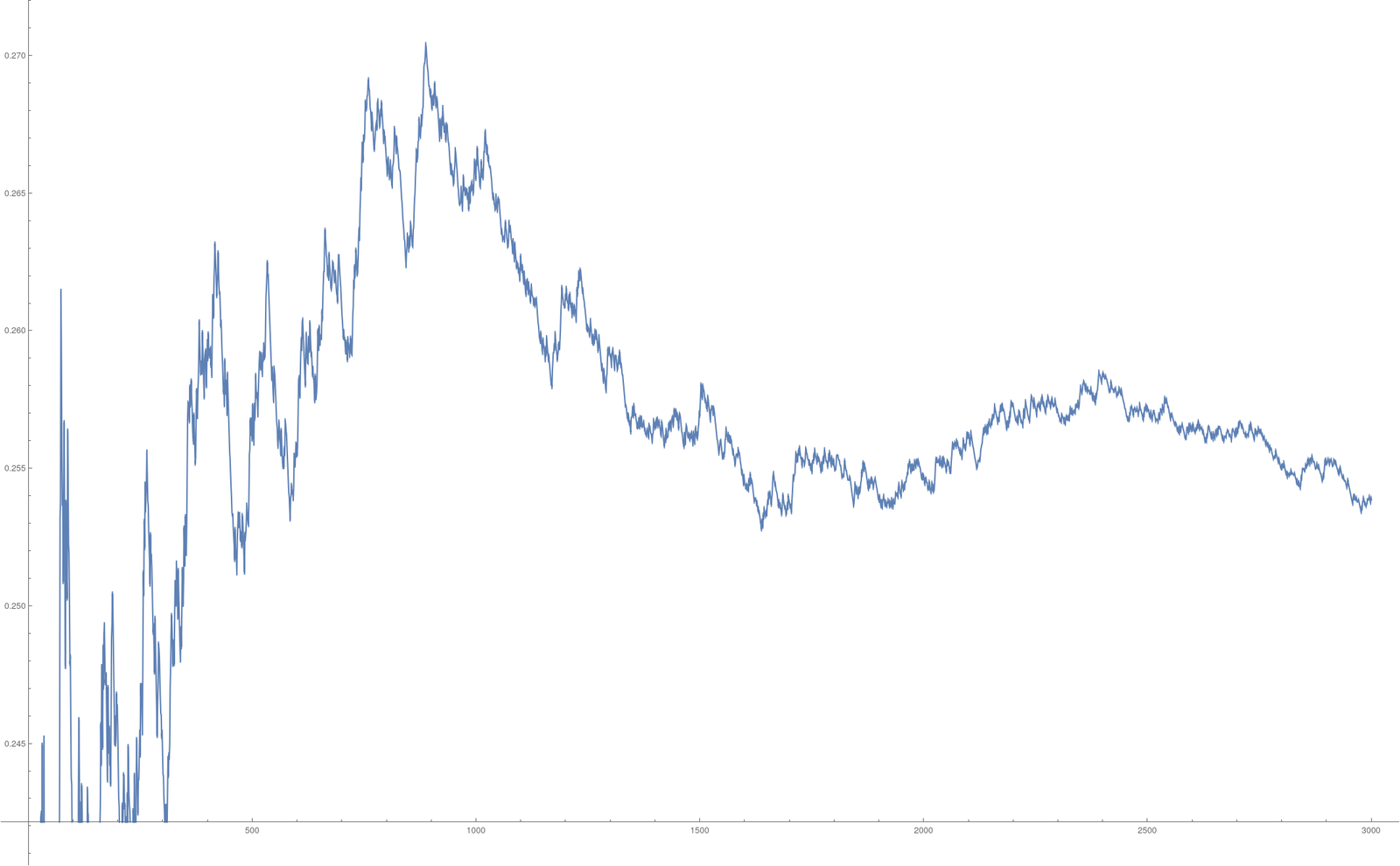# Data 04: Monte Carlo

All lecture, Data projects and Exam documets in one 313 page PDF
 The fourth Project (PDF) deals with integration.
The fourth and last project deals with Monte Carlo computations. This project is short. There are only 2 problems. The first is to use the digits of pi to integrate a function. The second is to estimate the area of the Mandelbrot set using Monte Carlo integration.

In the first part, we use the digits of π as a number generator. Lets illustrate this with taking 3 digit blocks. Here are 60 digits of π.
```3.14159265358979323846264338327950288419716939937510582097494
```
We can produce random numbers by building blocks of 3 to get the sequence
``` 141, 592, 653, 589, 793, 238, 462, 643, 383, 279,
502, 884, 197, 169, 399, 375, 105, 820, 974, 944
```
Now we get random numbers by dividing by 1000
```x1= 0.141, x2= 0.592 , x3=0.653   etc
```
Now, we can integrate a function like f(x)=x3 numerically by building the sum (∑k=120 (xk)^3)/20. In this case the sum is 0.2338 which is still a bit off from the 0.25 we want to have. But when using more digits this converges. For 600 digits, we get 0.244244, with 1000 digits we get 0.25467. Here is what we get depending on the number of digits. The k'th entry in this block uses 3k digits of π so that we have k numbers xk.In the second part, we shoot n randomly points into the box A given by { (x,y) with -2 ≤ x ≤ 1 and -1.5 ≤ y ≤ 1.5} and see how many times m we hit. The number (m/n)*box area = 9m/n is then an approximation of the Area of the Mandelbrot set. The Riemann integral would be completely inadequate here as the boundary of the Mandelbrot set is very complicated. Its dimension is even 2 and not 1 as for a curve (a result from 1991).

Here is the online python interpreter and here is the python code. Try to tune the parameters to get more accurate results!

 The following picture of the Mandelbrot set was produced with the Ray tracer Povray. You find the source code of a Povray program which produces a movie on this page. The program is so small that it could be tweeted. Here is the tweet and the movie.